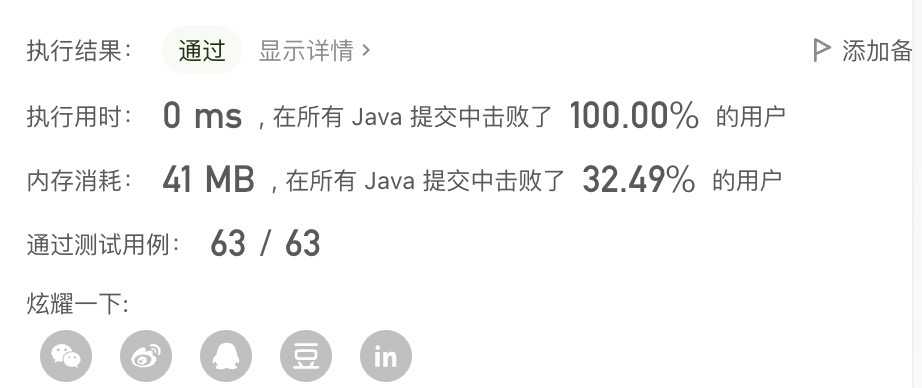> 文档中心 > LeetCode 每日一题——1022. 从根到叶的二进制数之和

# 1.题目描述

1022. 从根到叶的二进制数之和

``输入：root = [1,0,1,0,1,0,1]输出：22解释：(100) + (101) + (110) + (111) = 4 + 5 + 6 + 7 = 22``

``输入：root = 输出：0``

# 2.解题思路与代码

## 2.2 代码

``class Solution {    public int sumRootToLeaf(TreeNode root) { return process(root, 0);    }    public int process(TreeNode root, int ans) { if (root == null) {     return 0; } ans = ans * 2 + root.val; if (root.left == null && root.right == null) {     return ans; } int left = process(root.left, ans); int right = process(root.right, ans); return left + right;    }}``

## 2.3 测试结果# 3.总结

• 使用二叉树中序遍历
• 遍历的同时求当前节点的总和